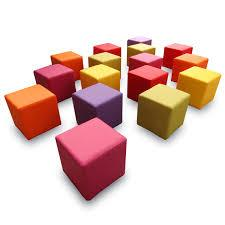# Decimetres 4163

Determine the length of the body and wall diagonals of the cube, the volume of which is equal to 0.343 decimetres. Also, calculate its surface.

u1 =  0.9899 dm
u2 =  1.2124 dm
S =  2.94 dm2

### Step-by-step explanation:Did you find an error or inaccuracy? Feel free to write us. Thank you!

Tips for related online calculators
The Pythagorean theorem is the base for the right triangle calculator.